# Statistical Tests¶

The Statistical tests cards allow you to make quantitative decisions by testing statistical hypotheses. Each card displays the outcome of a specific statistical test, and you can see more information about the test (e.g. what the test does, underlying assumptions, etc) by clicking the question icon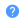in the card header.

The statistical tests cards are grouped into:

## One-sample tests¶

These types of tests allow you to compare the location parameters of a population to a hypothesized constant, or to compare the distribution of a population to a hypothesized one.

### Student t-test (one-sample)¶

A one-sample test to determine if the mean of a population is a specific value.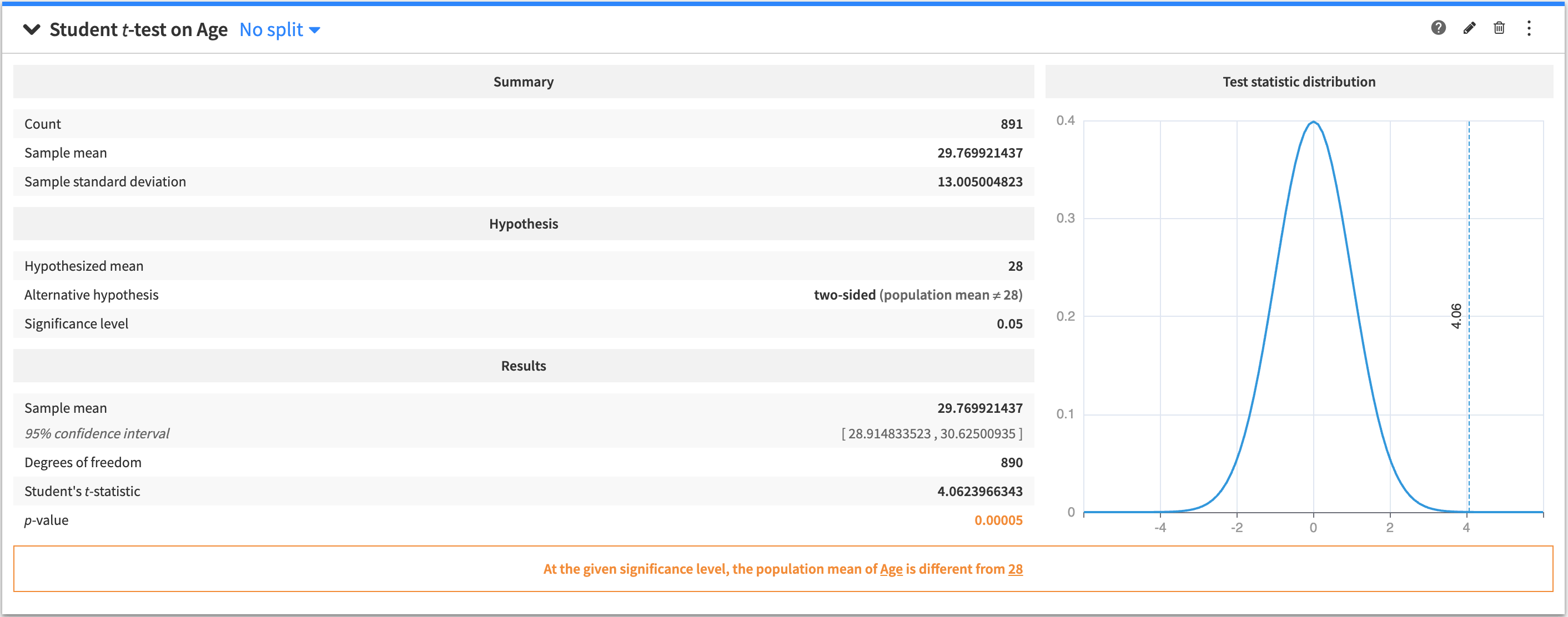To create this card, select a numerical (continuous) variable as the test variable, and specify a value for the hypothesized mean.

The output of the one-sample Student t-test contains:

• A summary of the test variable

• The tested hypothesis

• The results of the test

• A figure that displays the distribution of the test statistic.

• A conclusion about the test (whether the hypothesis is incorrect, or if the test is inconclusive)

### Sign test (one-sample)¶

A one-sample test to determine if the median of a population is a specified value.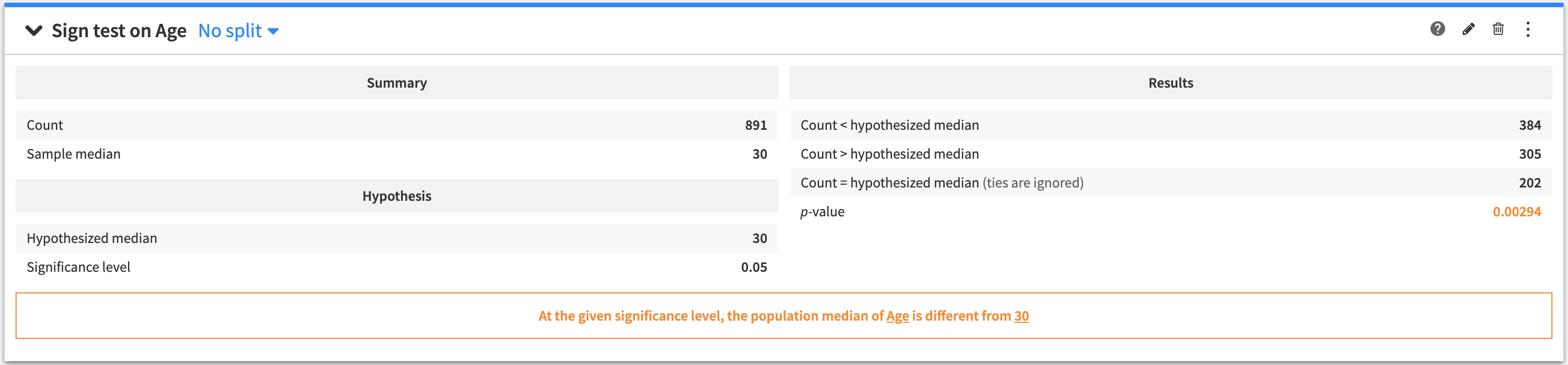To create this card, select a numerical (continuous) variable as the test variable, and specify a value for the hypothesized median.

The output of the one-sample Sign test contains:

• A summary of the test variable

• The tested hypothesis

• The results of the test

• A conclusion about the test (whether the hypothesis is incorrect, or if the test is inconclusive)

### Shapiro-Wilk test¶

A one-sample test to determine if a population is normally distributed.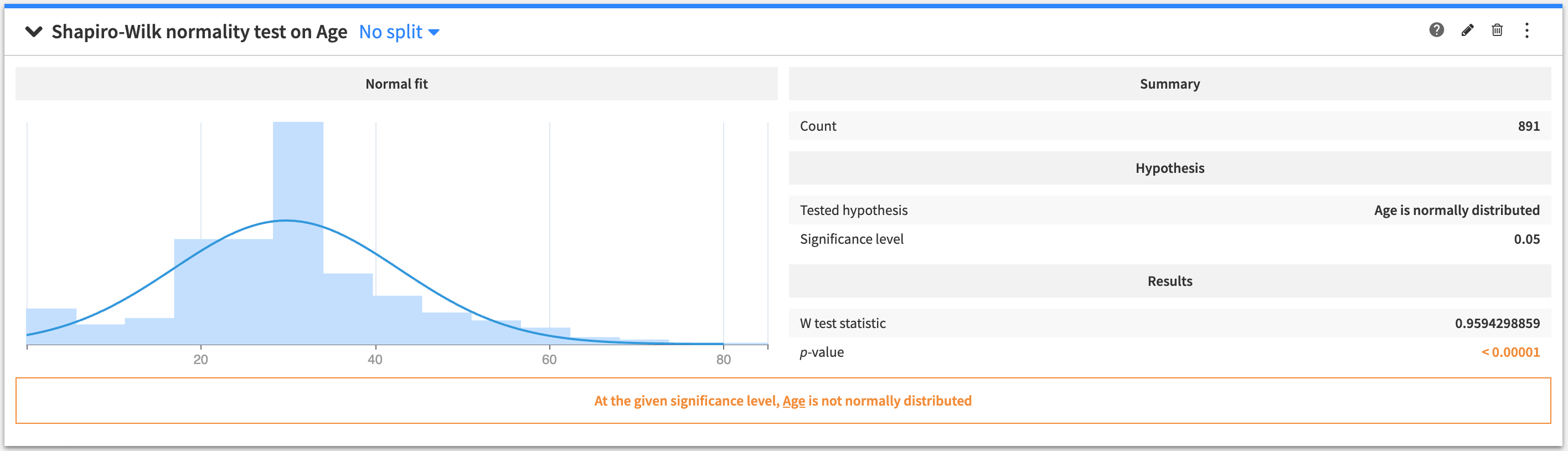To create this card, select a numerical (continuous) variable as the test variable. The tested hypothesis is that the sample comes from a normal (Gaussian) distribution.

The output of the Shapiro-Wilk test contains:

• A figure of a normal distribution fit to the data

• The summary of the data

• The tested hypothesis

• The results of the test

• A conclusion about the test (whether the hypothesis is incorrect, or if the test is inconclusive).

## Two-sample tests¶

These types of tests allow you to compare the location parameters of two populations, or to compare the distributions of two populations.

### Student t-test (two-sample)¶

A two-sample test to determine if the means of two populations are equal.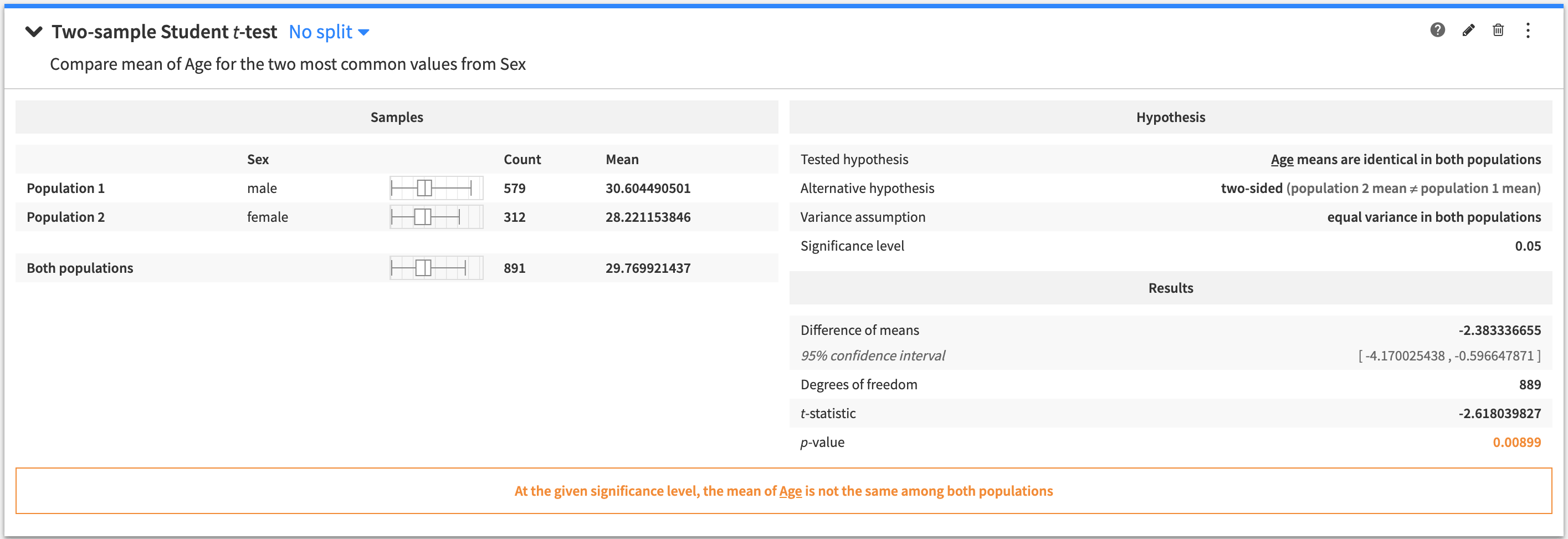To create this card:

• Select a numerical (continuous) variable as the “Test Variable”

• Select a categorical variable as the “Grouping Variable”

• Add values from the grouping variable to create two different populations “Population 1” and “Population 2”

The tested hypothesis is that the means of the two populations are identical.

The output of the two-sample Student t-test contains:

• A summary of the population samples

• The tested hypothesis

• The results of the test

• A conclusion about the test (whether the hypothesis is incorrect, or if the test is inconclusive).

### Median mood test (two-sample)¶

A two-sample test to determine if the medians of two populations are equal.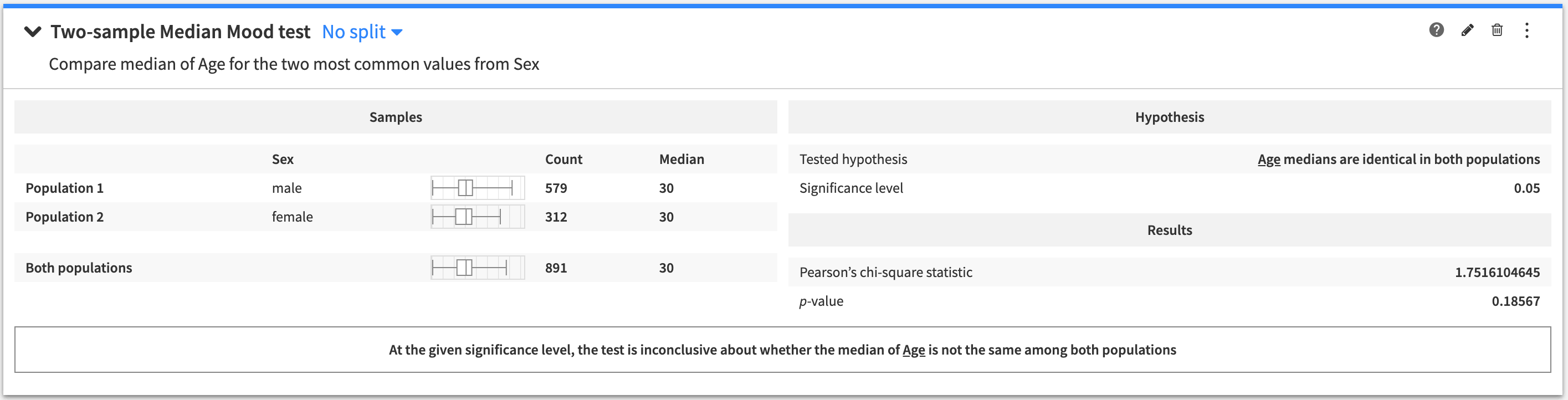To create this card:

• Select a numerical (continuous) variable as the “Test Variable”

• Select a categorical variable as the “Grouping Variable”

• Add values from the grouping variable to create two different populations “Population 1” and “Population 2”

The tested hypothesis is that the medians of the two populations are identical.

The output of the two-sample Median mood test contains:

• A summary of the population samples

• The tested hypothesis

• The results of the test

• A conclusion about the test (whether the hypothesis is incorrect, or if the test is inconclusive).

### Kolmogrov-Smirnov test (two-sample)¶

A two-sample test to determine if two populations are similarly distributed.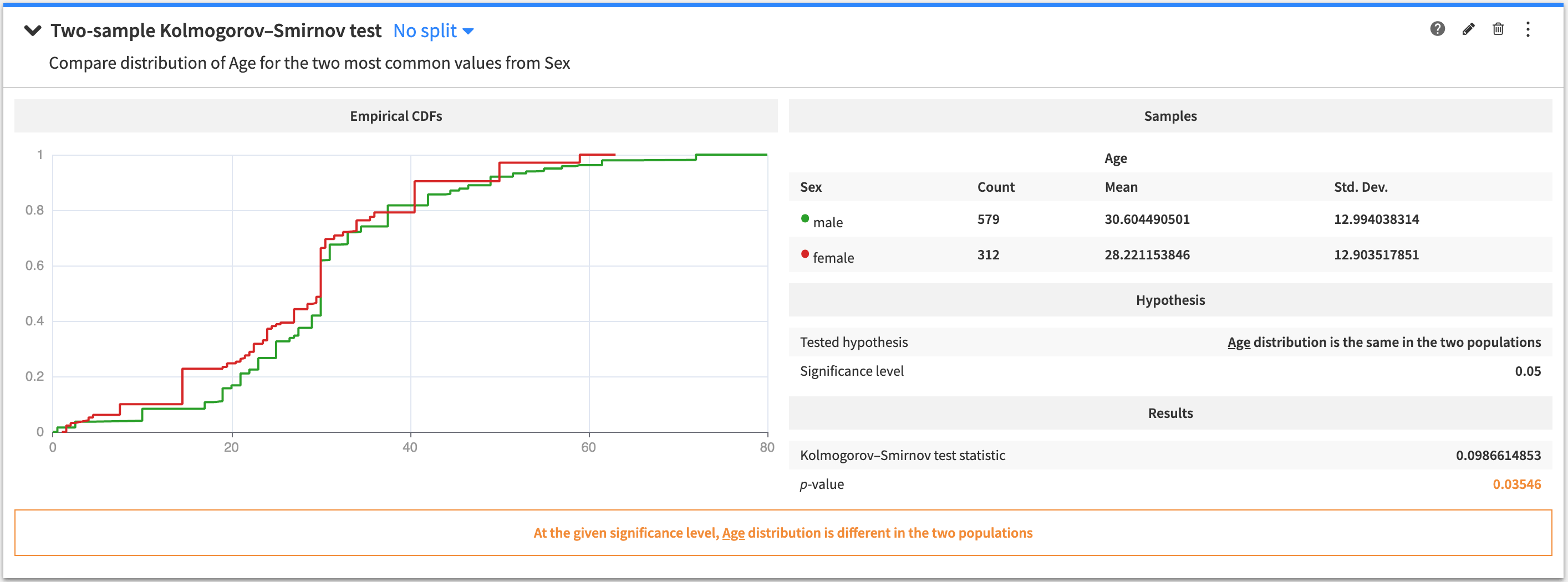To create this card:

• Select a numerical variable as the “Test Variable”

• Select a categorical variable as the “Grouping Variable”

• Add values from the grouping variable to create two different populations “Population 1” and “Population 2”

The tested hypothesis is that the probability distribution is the same in the two populations.

The output of the two-sample Kolmogorov-Smirnov test contains:

• A figure showing the empirical Cumulative Distribution Functions (CDFs) of the two populations

• A summary of the population samples

• The tested hypothesis

• The results of the test

• A conclusion about the test (whether the hypothesis is incorrect, or if the test is inconclusive).

## N-sample tests¶

These types of tests allow you to compare the location parameters of multiple populations.

### One-way ANOVA¶

An n-sample test to determine if the means of all populations are equal.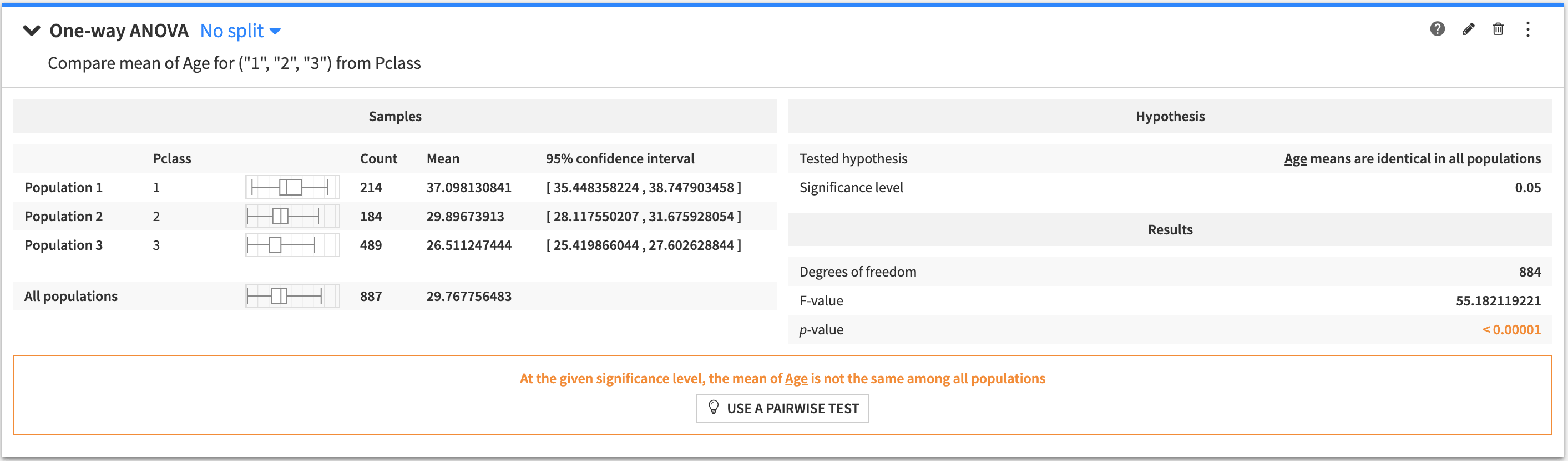To create this card:

• Select a numerical variable as the “Test Variable”

• Select a variable as the “Grouping Variable”

• Select either:

• “Build groups from most frequent values” and then specify a value for the “Maximum number of groups”, or

• “Define groups manually” and then enter the values of your grouping variable to form the population.

The tested hypothesis is that the mean of the test variable is identical in all populations.

The output of the One-way ANOVA test contains:

• A summary of the population samples in all the groups

• The tested hypothesis

• The results of the test

• A conclusion about the test (whether the hypothesis is incorrect, or if the test is inconclusive).

### Median mood test (N-samples)¶

An n-sample test to determine if the medians of all populations are equal.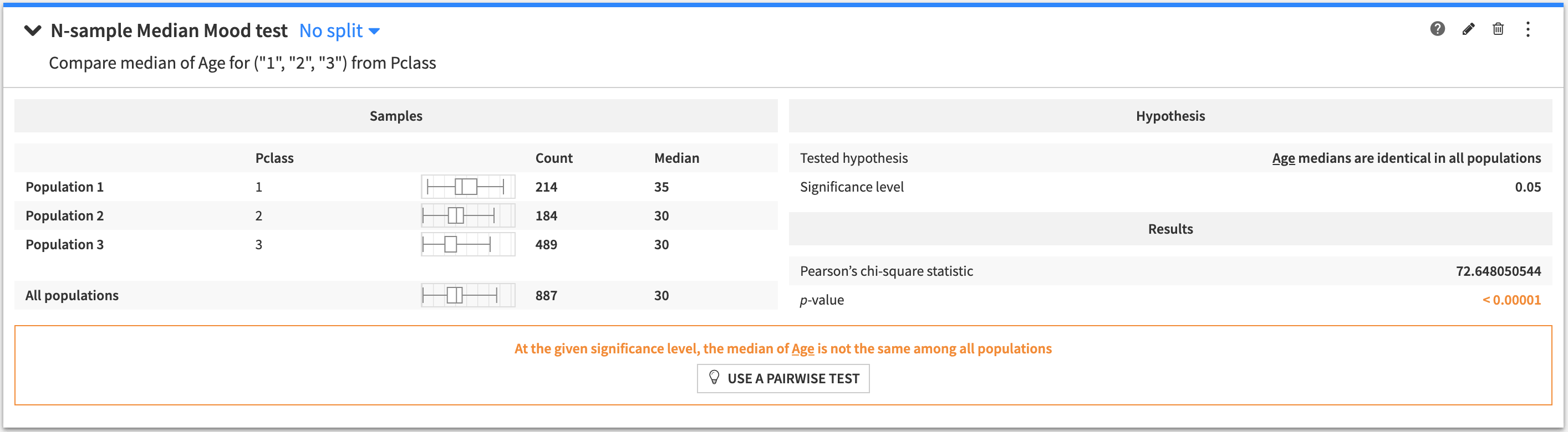To create this card:

• Select a numerical variable as the “Test Variable”

• Select a variable as the “Grouping Variable”

• Select either:

• “Build groups from most frequent values” and then specify a value for the “Maximum number of groups”, or

• “Define groups manually” and then enter the values of your grouping variable to form the population.

The tested hypothesis is that the median of the test variable is identical in all populations.

The output of the N-sample Median mood test contains:

• A summary of the population samples in all the groups

• The tested hypothesis

• The results of the test

• A conclusion about the test (whether the hypothesis is incorrect, or if the test is inconclusive).

### Pairwise student t-test¶

An n-sample test to determine if every pair of populations has the same mean.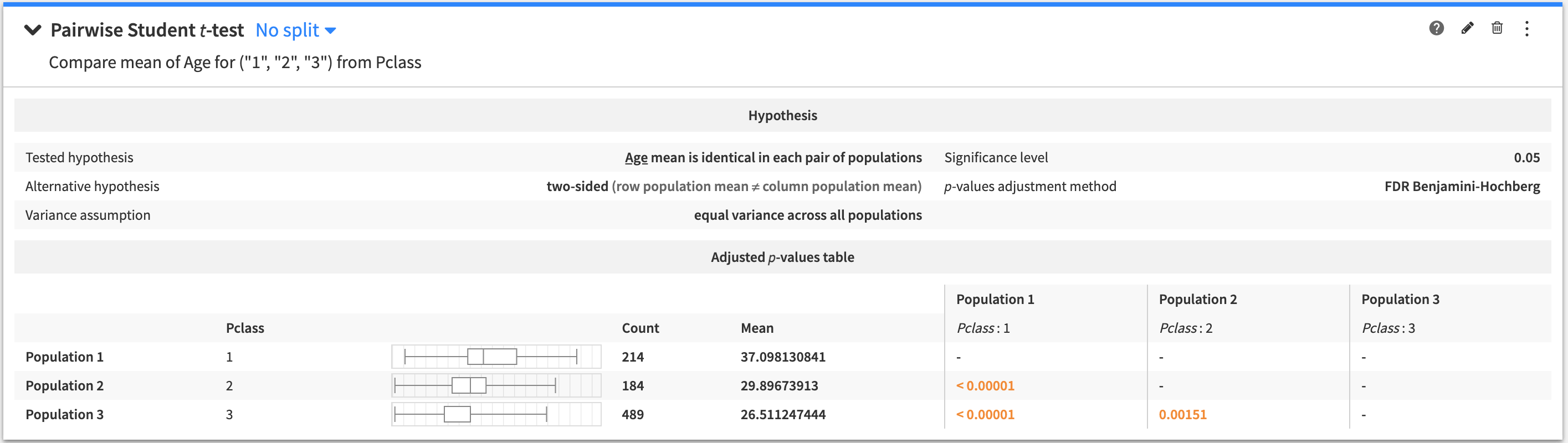To create this card:

• Select a numerical variable as the “Test Variable”

• Select a value for the “Adjustment Method” from the options: None, Bonferroni, and Holm-Bonferroni

• Select a variable as the “Grouping Variable”

• Select either:

• “Build groups from most frequent values” and then specify a value for the “Maximum number of groups”, or

• “Define groups manually” and then enter the values of your grouping variable to form the population.

The tested hypothesis is that the means of the paired populations are identical.

The output of the Pairwise t-test contains:

• The tested hypothesis

• A table of pairwise p-values. Holding the cursor over any given p-value tells you whether the hypothesis is rejected, or if the test is inconclusive.

### Pairwise median mood test¶

An n-sample test to determine if every pair of populations has the same median.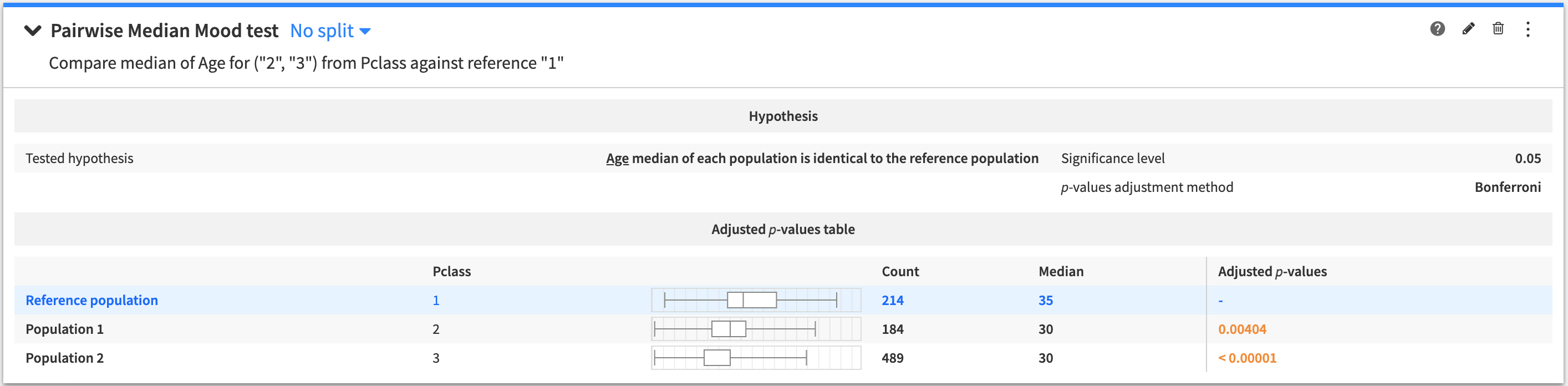To create this card:

• Select a numerical variable as the “Test Variable”

• Select a value for the “Adjustment Method” from the options: None, Bonferroni, and Holm-Bonferroni

• Select a variable as the “Grouping Variable”

• Select either:

• “Build groups from most frequent values” and then specify a value for the “Maximum number of groups”, or

• “Define groups manually” and then enter the values of your grouping variable to form the population.

The tested hypothesis is that the medians of the paired populations are identical.

The output of the Pairwise median mood test contains:

• The tested hypothesis

• A table of pairwise p-values. Holding the cursor over any given p-value tells you whether the hypothesis is rejected, or if the test is inconclusive.

## Categorical test¶

This type of test determines whether there is a significant relationship between two categorical variables in a sample or if the two variables are independent.

### Chi-square independence test¶

A test to determine if two categorical variables are independent.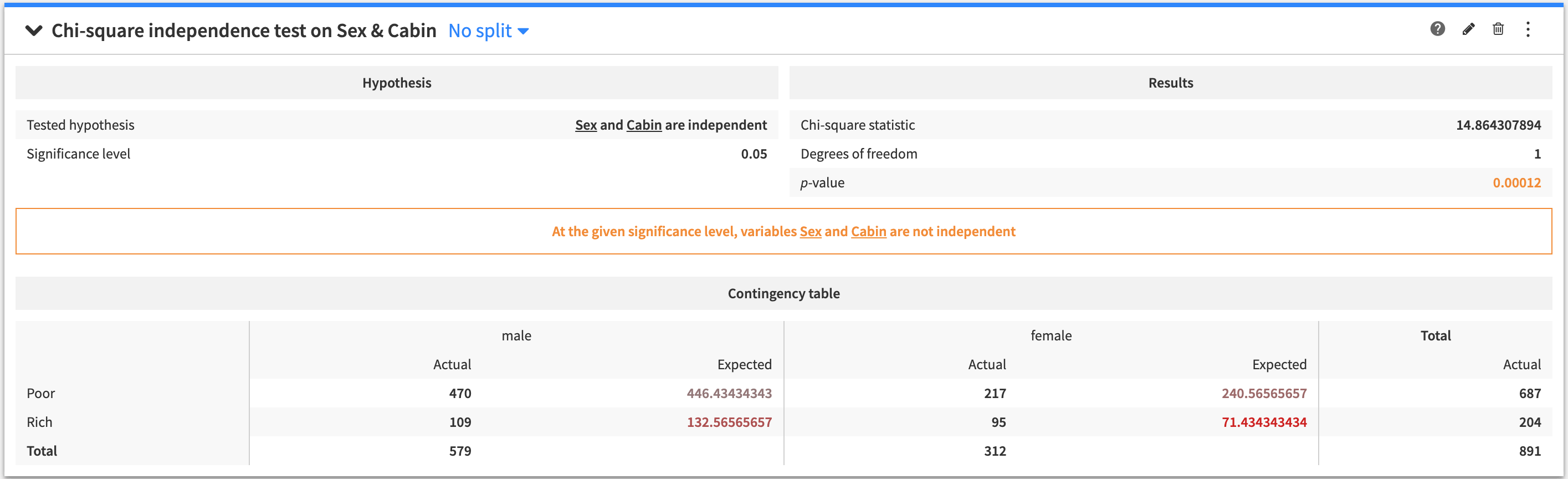To create this card:

• Select categorical variables for “Variable 1” and “Variable 2”

• Specify numerical values for the “Maximum X Values to Display” and the “Maximum Y Values to Display”

The tested hypothesis is that the two variables are independent.

The output of the Chi-square independence test contains:

• The tested hypothesis

• The results of the test

• A conclusion about the test (whether the hypothesis is incorrect, or if the test is inconclusive)

• A table of the actual versus expected number of observations for each row and column combination in the table count of values for each cell